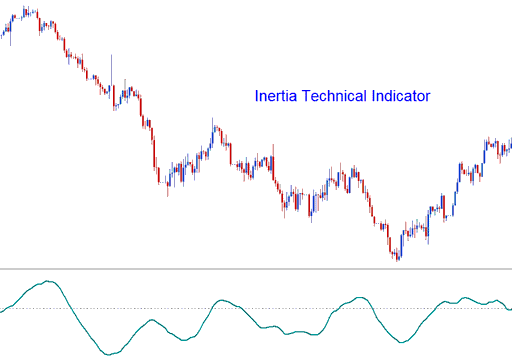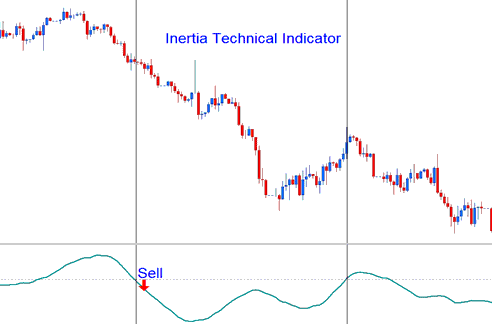# Inertia Indices Technical Analysis and Inertia Indices Trading Signals

Developed by Donald Dorsey and was originally used to trade Stocks & Commodities market, before indices traders took it and started trading the stock indexes trading market using this indicator.

Dorsey chose to name it "Inertia" because of his interpretation of the stock indexes trading market trend. He claimed that a stock indices market trend is the overall result of inertia and thus it takes more momentum for a trending market to reverse its direction than to continue moving in the same direction. Therefore, a indices trend is the measurement of market inertia. This is an oscillator that uses the scale of zero to a hundred. Signals are generated using the 50 level center line crossover method.In physics, the term Inertia is defined in terms of mass & direction of motion. Using the standard technical analysis, the direction of motion of the indices trend can be easily defined. However, the mass cannot be easily defined. Dorsey claimed that the volatility of a financial instrument may be the simplest & the most accurate measurement of inertia. This theory led to the use of the Relative Volatility Index (RVI) as the basis to be used as a indices trend indicator. Therefore Inertia is comprised of: RVI smoothed by a linear regression.

## Indices Technical Analysis and Generating Indices Trading Signals

When it comes to trading the stock indexes trading market using this indicator, the signals generated are fairly simple to interpret. Below are two examples illustrated using indices charts showing how buy and sell stock indices signals are generated using Inertia.

If the Inertia is above 50, positive inertia is indicated, this therefore defines the long-term indices trend as upward as long as the indicator remains above 50. When it crosses to levels below 50 then this is interpreted as an exit signal. The chart below shows an example of how a buy stock indices signal is generated.Bullish Signal

## Bearish Sell Indices Trading Signal

If the Inertia is below 50, negative inertia is indicated, this therefore defines the long-term indices trend as downwards as long as the indicator remains below 50. If it goes above 50 then this is interpreted as an exit signal. The stock indices chart below shows how a sell stock indices signal generated.Bearish Signal